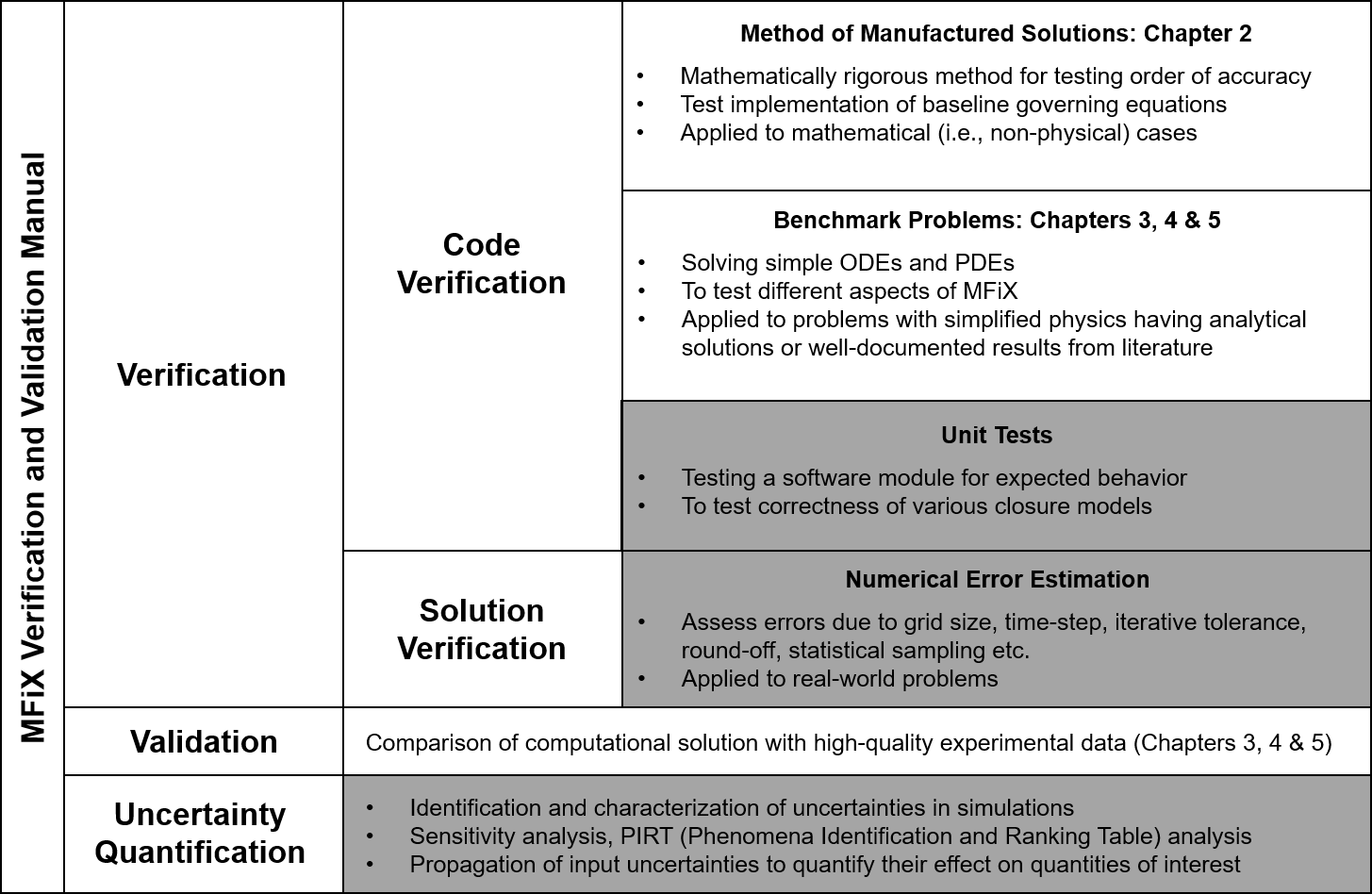# 1. Introduction¶

The MFIX Verification and Validation Manual, referred to herein as the V&V Manual, aims to document verification tests and validation cases for the MFIX suite. Additionally, this manual attempts to capture best practices for V&V as part of a broader approach to software quality assurance (SQA). The goal is to perform a systematic verification of features available in the code for correctness and numerical accuracy. Future work will include validation cases to assess the suitability of the physical models implemented within MFIX. The V&V Manual also serves as a guide for periodic and automated testing of the software by the developers.

## 1.1. Verification and Validation¶

For the purpose of this manual, the terms verification and validation are defined as follows 

• Verification: The process of determining that a numerical model implementation accurately represents the developer’s conceptual description of the model and the mathematical solution to the model.

• Validation: The process of determining the degree to which a model is an accurate representation of the real world from the perspective of the intended uses of the model.

Roache  succinctly describes verification as an assessment on “solving the equations right” whereas validation is “solving the right equations.” Fig. 1.1 illustrates the relationship between verification and validation processes schematically.Fig. 1.1 Verification and validation process .

Verification deals with the mathematics of the simulation and involves assessing the correctness of the computer code and numerical algorithms (i.e., code verification) as well as the accuracy of the numerical solution (i.e., solution verification). Validation deals with the physics of the problem and assesses whether the selected mathematical model satisfactorily predicts the physics of interest. Roache  further noted the distinction between verification and validation as, “Verification is a mathematics issue; not a physics issue.” Hence, verification precedes validation.

## 1.2. Verification Test Selection Criteria¶

Verification test cases are selected based upon the following criteria:

• Each test case should exercise one or more sub-models for a physical phenomenon

• Simulations must be computationally inexpensive to facilitate regular testing

• There should be minimal overlap between tests

The preference and thereby quality of a test is assessed on the following criteria:

• (Most preferable) Examination of numerical error between the exact solution and the numerical solution for problems where an analytical solution is available (i.e., verification using method of exact solutions) or a manufactured solution is obtainable

• (Less preferable) Comparison of numerical solutions to established results in literature (i.e., similar to validation, but using benchmark problems)

• (Least preferable) Comparison of numerical solutions to results obtained from previous versions of the same code (i.e., regression test) or from another verified code (i.e., code comparison)

## 1.3. Testing Frequency¶

Cases presented within this document are tested at various intervals as part of the SQA process. Before 2014, code integrity in MFIX was tracked through a series of nightly regression tests based on an open source software testing framework called QMTest, which provided a single snapshot on a daily basis . This method was replaced by a continuous integration (CI) server for greater testing and archival flexibility.

Cases that execute quickly are tested whenever modifications are committed to the source code repository to quickly detect any issues generated by the changes. However, computationally burdensome verification and validation cases are tested less frequently to prevent overwhelming the CI server. A summary table at the start of each chapter indicates the frequency at which each case is tested.

## 1.4. Case files and datasets¶

Case files and datasets for the tests outlined in this document are provided with the MFIX source code under the mfix/tests directory. All presented data are representative of results from the current MFIX release unless explicitly noted.

## 1.5. Organization¶

Chapter 2 provides verification tests that use the Method of Manufactured Solution (MMS) to determine whether the observed order of error reduction with grid refinement matches the formal order. Order testing with MMS is considered to be a very rigorous procedure for code verification.

Chapter 3 provides verification and validation test cases using MFiX Fluid Model which represents the framework for solving gas-phase equations used in Two-Fluid Model (MFiX-TFM), Discrete Element Model (MFiX-DEM) and Particle-In-Cell (MFiX-PIC) model. Chapter 4 provides examples of verification problems using MFiX-DEM while verification and validation test cases using MFiX-PIC are presented in Chapter 5. Fig. 1.2 shows the scope of the V&V activity covered in this manual.Fig. 1.2 Scope of MFIX verification and validation activity covered in this manual. (Greyed parts indicate future or ongoing activities not presented in the current version of this document.)